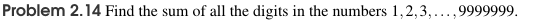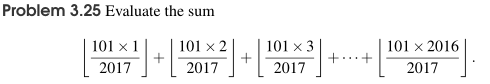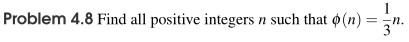# Topics Covered in Math Challenge III

The Math Challenge III class has more focus on problem-solving techniques rather than fundamental concepts and knowledge points. It is assumed that the students have learned the fundamentals. That being said, basic knowledge points are still covered if deemed necessary by the instructor, according to the students' level of experiences.

The order of these topics may vary, and these topics might not be all covered. The coverage will depend on time schedule and overall progress.

### Algebra

The answer key for MC III Algebra is available by clicking here.

#### Chapter Topics

• Review of Logarithms
• Fundamentals of Complex Numbers
• Techniques for Solving Equations
• Techniques for Solving Inequalities

#### Sample Questions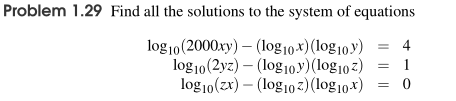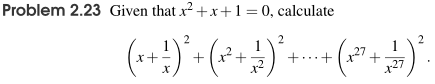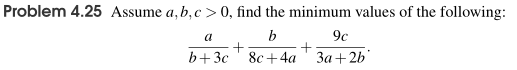### Geometry

The answer key for MC III Geometry is available by clicking here.

#### Chapter Topics

• Geometry with Algebraic Techniques
• Geometric Transformations
• Transformations Continued
• Trigonometry
• Menelaus and Ceva

#### Sample Questions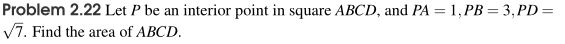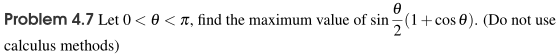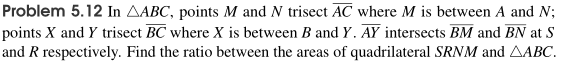### Combinatorics

The answer key for MC III Combinatorics is available by clicking here.

#### Chapter Topics

• Principles in Combinatorics
• Problem Types and Techniques
• Binomial Theorem and Combinatorial Identities
• Bijection and Review Problems
• Probability - The Classical Model
• Geometric Probability and Random Variables

#### Sample Questions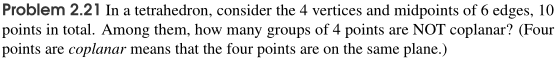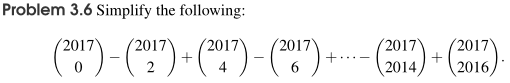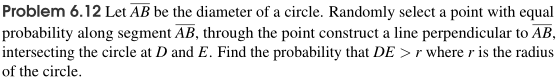### Number Theory

The answer key for MC III Number Theory is available by clicking here.

#### Chapter Topics

• Review of Fundamental Concepts, Methods, and Theorems
• Problem Solving Techniques in Number Theory
• The Floor Function
• Number Theory Functions
• Further Practice in Number Theory

#### Sample Questions